模型评估——定量分析预测的质量

1. 评分方法：评估者具有score()，面向其要解决的问题，可以提供一个默认评估标准。 这部分内容不在本文中讲述，因为它因不同的模型而异，所以会在各个模型的介绍文档中讲述。
2. 评分参数：使用交叉验证的模型评估工具（如model_selection.cross_val_scoremodel_selection.GridSearchCV）依赖于内部评分策略。这些内容会在其他章节（评分参数：定义模型评判规则）中讨论
3. 度量函数：metrics模块实现了针对特定目的评估误差的功能。 这些指标会在下面这些章节详细介绍：Classification metrics, Multilabel ranking metrics, Regression metrics and Clustering metrics.
最后，Dummy estimators对于获得随机预测的这些指标的基准值是有用的。

1. 本文译自Python库sklearn里的官方文档
2. 需要读者对Python有一定的了解
3. 本文超长…而且因为水平有限，有些地方翻译的较为生硬。

评分参数：定义模型评价规则

模型选择和评估通常使用工具（比如model_selection.GridSearchCVmodel_selection.cross_val_score），使用一个scoring参数控制那个被我们应用在我们的评价系统的度量方法。

公共案例：预定义值

对于最常见的用例，您可以指定一些自带scoring参数的评分对象; 下表显示了所有可能的值。 所有得分手对象遵循惯例：返回值越大，分数越高，模型越优。 因此，衡量模型和数据之间距离的度量（如metrics.mean_squared_error）可以用它们的负值作为评分对象的返回值。

Scoring Function Comment

‘accuracy’ metrics.accuracy_score
‘average_precision’ metrics.average_precision_score
‘f1’ metrics.f1_score
‘f1_micro’ metrics.f1_score
f1_macro’ metrics.f1_score
‘f1_weighted’ metrics.f1_score
‘f1_samples’ metrics.f1_score
‘neg_log_loss’ metrics.log_loss
‘precision’ etc metrics.precision_score
‘recall’ etc metrics.recall_score
‘roc_auc’ metrics.roc_aur_score

‘adjusted_mutual_info_score’ metrics.adjusted_mutual_info_score
‘adjusted_rand_score’ metrics.adjusted_rand_score
‘completeness_score’ metrics.completeness_score
‘fowlkes_mallow s_score’ metrics.fowlkes_mallows_score
‘homogeneity_score’ metrics.homogeneity_score
‘mutual_info_score’ metrics.mutual_info_score
‘normalized_mutual_info_score’ metrics.normalized_mutual_info_score
‘v_measure_score’ metrics.v_measure_score

‘explained_variance’ metrics.explained_variance_score
‘neg_mean_absolute_error’ metrics.mean_absolute_error
‘neg_mean_squared_error’ metrics.mean_squared_error
‘neg_mean_squared_log_error’ metrics.mean_squared_log_error
‘neg_median_absolute_error’ metrics.median_absolute_error
‘r2’ metrics.r2_score

from sklearn import svm, datasets  # 导入SVM模型库和数据集库
from sklearn.model_selection import cross_val_score  # 导入模型选择库中的交叉验证分数函数
'''加载鸢尾花数据集'''
X, y = iris.data, iris.target
clf = svm.SVC(probability=True, random_state=0)  # 创建SVC分类器
'''输出分类器的评分'''
score_arr = cross_val_score(clf, X, y, scoring='neg_log_loss')
print score_arr  # 输出 [-0.0757138  -0.16816241 -0.07091847]
print score_arr.shape  # 输出 (3L,)

model = svm.SVC()  # 在创建一个SVC分类器
'''应用另一种不存在的评分方式'''
cross_val_score(model, X, y, scoring='wrong_choice')  # 会报错ValueError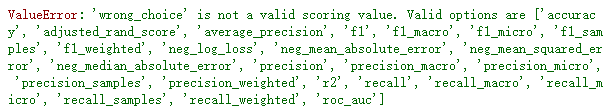PS：ValueError异常列出的值对应于以下部分中描述的测量预测精度的函数。 这些评分对象存储在字典sklearn.metrics.SCORERS中。

根据度量函数定义你的评分策略

模块sklearn.metrics还展示了一组测量预测误差（给出了真实值和预测值）的简单函数：

• _score结尾的函数返回值用于最大化，越高越好
• _error_loss结尾的函数返回一个值用于最小化，越低越好。当使用make_scorer()函数将其转换为评分对象时，请将greater_is_better参数设置为False（默认为True）。

许多指标不会以scoring为名称，有时是因为它们需要其他参数，例如fbeta_score。在这种情况下，您需要生成一个适当的scorer对象。生成可评估对象进行评分的最简单的方法是使用make_scorer()函数。该函数将度量转换为可用于模型评估的可调用的数据类型（callable）。
一个典型的用例是使用无默认值的参数转换包装库中已存在的度量函数，例如fbeta_score()函数的beta参数：

from sklearn.metrics import fbeta_score, make_scorer  # 导入fbeta评分函数和一个转换函数
ftwo_scorer = make_scorer(fbeta_score, beta=2)  # 通过make_scorer创建新方法
from sklearn.model_selection import GridSearchCV
from sklearn.svm import LinearSVC
'''待评价的分类模型是线性SVC，超参数域为{1， 10}， 评分方法为前面新转换的方法'''
grid = GridSearchCV(LinearSVC(), param_grid={'C': [1, 10]}, scoring=ftwo_scorer)

第二个用例是使用make_scorer将一个简单的python函数构建一个完全自定义的scorer对象，它可以使用几个参数：

• 你要使用的python函数（在下面的示例中为my_custom_loss_func()
• 明确python函数是否返回一个分数（greater_is_better = True，默认值）或一个损失值（greater_is_better= False）。 如果一个损失，得分器对象的python函数的输出被取负值以符合交叉验证惯例，更优的模型返回更高的值。
• 仅用于分类度量的时候：判断您提供的python函数是否需要连续性判断（needs_threshold = True）。 默认值为False。
• 任何其他参数，如f1_score()函数中的参数：betalabels

import numpy as np
def my_custom_loss_func(ground_truth, predictions):
"""
自定义损失函数——其实就是典型的SVM损失函数
groud_truth: 真实值
predictions: 预测值
"""
diff = np.abs(ground_truth - predictions).max()
return np.log(1 + diff)
loss  = make_scorer(my_custom_loss_func, greater_is_better=False)  # 创建为scorer
score = make_scorer(my_custom_loss_func, greater_is_better=True)
ground_truth = [, ]
predictions  = [0, 1]
from sklearn.dummy import DummyClassifier
clf = DummyClassifier(strategy='most_frequent', random_state=0)  # 创建简陋分类模型
clf = clf.fit(ground_truth, predictions)  # 训练模型
loss(clf,ground_truth, predictions)  # 损失值，False：取负

score(clf,ground_truth, predictions)  # 评分， True：取正

1. 自定义评分函数时，需要用到真实值和预测值，然后根据自己的想法输出预测的误差或者分值，这里输出的是误差。
2. make_scorer()函数的参数greater_is_better是为了适应灵活的自定义评分函数的。当你的评分函数输出损失值的时候，参数为False，即对损失值取负；输出分值的时候，反之。这都是为了遵从前面所说的越大越优的惯例

应用你自己的评分对象

您可以通从头开始构建自己的评分对象，而不使用make_scorer()工厂函数，这样生成的scorer模型更灵活。 要成为scorer，需要符合以下两个规则所指定的协议：

• 可以使用参数(estimator，X，y)调用，其中estimator是应该评估的模型，X是验证数据，y是X（在监督的情况下）的真实标签或None（在无监督的情况下）。
• 它返回一个浮点数，用于量化X上的estimator参考y的预测质量。 再次，按照惯例，更高的值表示更好的预测模型，所以如果你的返回的是损失值，应取负。

使用多种度量指标

Scikit-learn还允许在GridSearchCVRandomizedSearchCVcross_validate中使用多个度量指标。为评分参数指定多个评分指标有两种方法：

• 作为一个包含字符串的迭代器
scoring = ['accuracy', 'precision']
• 作为一个scorer名字到scorer函数的映射
from sklearn.metrics import accuracy_score
from sklearn.metrics import make_scorer
scoring = {'accuracy': make_scorer(accuracy_score),
'prec': 'precision'}

请注意，字典中的值可以是scorer函数，也可以是一个预定义的度量指标的字符串。目前，只有那些返回单一分数的scorer函数才能在dict内传递。 不允许返回多个值的Scorer函数，并且需要一个包装器才能返回一个度量：

from sklearn.model_selection import cross_validate  # 交叉验证函数
from sklearn.metrics import confusion_matrix
X, y = datasets.make_classification(n_classes=2, random_state=0)  # 一个简陋的二分类数据集
svm = LinearSVC(random_state=0)
def tp(y_true, y_pred): return confusion_matrix(y_true, y_pred)[0, 0]
def tn(y_true, y_pred): return confusion_matrix(y_true, y_pred)[0, 0]
def fp(y_true, y_pred): return confusion_matrix(y_true, y_pred)[1, 0]
def fn(y_true, y_pred): return confusion_matrix(y_true, y_pred)[0, 1]
scoring = {'tp' : make_scorer(tp), 'tn' : make_scorer(tn),
'fp' : make_scorer(fp), 'fn' : make_scorer(fn)}
cv_results = cross_validate(svm.fit(X, y), X, y, scoring=scoring)
print(cv_results['test_tp'])  # 获取test数据集的tp值 [12 13 15]
print(cv_results['test_fn'])  # 获取test数据集的fn值 [5 4 1]

分类度量

sklearn.metrics模块实现了几种损失函数、评分函数和功能函数来测量分类性能。 某些指标可能需要正例，置信度值或二进制决策值的概率估计。 大多数指标应用的是：通过sample_weight参数，让每个样本为总分提供加权贡献。

precision_recall_curve(y_true, probas_pred)
roc_curve(y_true, y_score[, pos_label, …])

cohen_kappa_score(y1, y2[, labels, weights, …])
confusion_matrix(y_true, y_pred[, labels, …])
hinge_loss(y_true, pred_decision[, labels, …])
matthews_corrcoef(y_true, y_pred[, …])

accuracy_score(y_true, y_pred[, normalize, …])
classification_report(y_true, y_pred[, …])
f1_score(y_true, y_pred[, labels, …])
fbeta_score(y_true, y_pred, beta[, labels, …])
hamming_loss(y_true, y_pred[, labels, …])
jaccard_similarity_score(y_true, y_pred[, …])
log_loss(y_true, y_pred[, eps, normalize, …])
precision_recall_fscore_support(y_true, y_pred)
precision_score(y_true, y_pred[, labels, …])
recall_score(y_true, y_pred[, labels, …])
zero_one_loss(y_true, y_pred[, normalize, …])

dcg_score(y_true, y_score[, k])
ndcg_score(y_true, y_score[, k])

average_precision_score(y_true, y_score[, …])
roc_auc_score(y_true, y_score[, average, …])

从二分类到多分类多标签

一些度量基本上是针对二进制分类任务（例如f1_scoreroc_auc_score）定义的。在这些情况下，假设默认情况下，正类标记为1（尽管可以通过pos_label参数进行配置），默认情况下仅评估正标签。
将二进制度量扩展为多类或多标签问题时，数据将被视为二分类问题的集合，即一对多类型。下面是综合二分类度量的值的多种方法，不同的方法可能适用于不同的情况，通过参数average来选择：

• "macro"简单地计算二分类度量的平均值，赋予每个类别相同的权重。
• "weighted"计算的是每个二分类度量的加权平均。
• "micro"，每个二分类对总体度量的贡献是相等的（除了作为样本权重的结果）。
• "sample"仅适用于多标签问题。它不计算每个类别的度量，而是计算评估数据中每个样本的真实和预测类别的度量，并返回（sample_weight-weighted）平均值。
• 选择average = None将返回每个类的分值。

多标签数据用于指标评估时，也像二分类的标签传入一个类别标签的数组，传入的是一个标签矩阵，元素ij在样本i的标签是j的时候取值为1，否则为0。

精确度

accuracy_score函数用于计算预测的精度。在多标签分类中，函数返回子集精度。 如果样本的整套预测标签与真正的标签组一致，则子集精度为1.0; 否则为0.0。如果y^i$\hat {y} _i$是第i个样本的预测值，yi$y_i$是相应的真实值，则nsamples$n_ \text {samples}$上的精度为：
accuracy(y,y^)=1nsamplesnsamples1i=01(y^i=yi)$\text{accuracy}(y, \hat{y}) = \frac{1}{n_\text{samples}}\sum_{i=0}^{n_\text{samples}-1} 1(\hat{y}_i = y_i)$

import numpy as np
from sklearn.metrics import accuracy_score
y_pred = [0, 2, 1, 3]
y_true = [0, 1, 2, 3]
accuracy_score(y_true, y_pred)  # 用比值的方式输出精度 输出：0.5
accuracy_score(y_true, y_pred, normalize=False)  # 用计数的方式输出精度 输出：2
'''多标签的情况'''
accuracy_score(np.array([[0, 1], [1, 1]]), np.ones((2, 2)))  # 输出 0.5

Cohen’s kappa

函数cohen_kappa_score()计算Cohen’s kappa统计量。这个方法是想比较不同的人表计的正确率，而非针对分类器。kappa分数是-1和1之间的数字。0.8以上的分数通常被认为是不错的结果; 零或更低表示没有效果（就像瞎蒙的一样）。
Kappa分数可用于二分类问题或多分类问题，但不能用于多重标签问题。

from sklearn.metrics import cohen_kappa_score
y_true = [2, 0, 2, 2, 0, 1]
y_pred = [0, 0, 2, 2, 0, 2]
cohen_kappa_score(y_true, y_pred)  # 输出0.4285...

混乱矩阵

confusion_matrix()函数通过计算混乱矩阵来评估分类精度。根据定义，元素ij表示：真实值为i，预测值为j的情况的个数，例子如下：

from sklearn.metrics import confusion_matrix
y_true = [2, 0, 2, 2, 0, 1]
y_pred = [0, 0, 2, 2, 0, 2]
confusion_matrix(y_true, y_pred)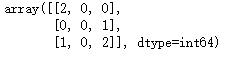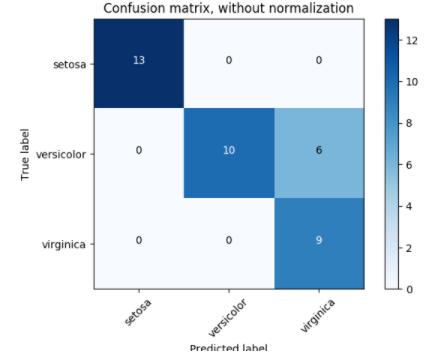y_true = [0, 0, 0, 1, 1, 1, 1, 1]
y_pred = [0, 1, 0, 1, 0, 1, 0, 1]
'''t表示正例，f表示反例，n表示预测错误，p表示预测正确'''
tn, fp, fn, tp = confusion_matrix(y_true, y_pred).ravel()
tn, fp, fn, tp  # 输出 (2, 1, 2, 3)

分类报告

函数classification_report()创建了一个展示主要分类度量指标的文本报告，这是一个使用自定义target_name和内部标签的小例程。

from sklearn.metrics import classification_report
y_true = [0, 1, 2, 2, 0]
y_pred = [0, 0, 2, 1, 0]
target_names = ['class 0', 'class 1', 'class 2']
cls_rpt = classification_report(y_true, y_pred, target_names=target_names)
print cls_rpt
print type(cls_rpt)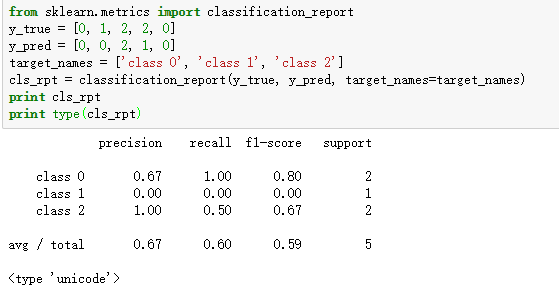汉明损失

函数hamming_loss()计算两组样本之间的平均汉明损失或汉明距离。如果y^j$\hat{y} _j$是给定样本的第j个标签的预测值，则yj$y_j$是相应的真实值，而nlabels$n_ \text {labels}$是类或标签的数量，则两个样本之间的汉明损失Lhamming$L_ { hamming}$定义为：
LHamming(y,y^)=1nlabelsnlabels1j=01(y^jyj)$L_{Hamming}(y, \hat{y}) = \frac{1}{n_\text{labels}} \sum_{j=0}^{n_\text{labels} - 1} 1(\hat{y}_j \not= y_j)$

from sklearn.metrics import hamming_loss
y_pred = [1, 2, 3, 4]
y_true = [2, 2, 3, 4]
hamming_loss(y_true, y_pred)  # 二分类单一标签 输出 0.25
hamming_loss(np.array([[0, 1], [1, 1]]), np.zeros((2, 2)))  # 二分类多标签 输出：0.75

Jaccard 相似性相关系数

jaccard_similarity_score()函数计算标签集对之间的平均（默认）或Jaccard相似系数的总和，也称为Jaccard索引。设真实标签集yi$y_i$和预测标签集合y^i$\hat {y} _i$，将第i个样本的Jaccard相似系数定义为
Jyiy^i=|yiy^i||yiy^i|$J（y_i，\hat {y} _i）= \frac {| y_i \cap \hat {y} _i |} {| y_i \cup \hat {y} _i |}$

import numpy as np
from sklearn.metrics import jaccard_similarity_score
y_pred = [0, 2, 1, 3]
y_true = [0, 1, 2, 3]
jaccard_similarity_score(y_true, y_pred)  # 输出 0.5
jaccard_similarity_score(y_true, y_pred, normalize=False)  # 输出 0.75

准确率、召回率和F度量

直观地说，准确率(presicion)是分类器不错判的能力，并且召回率(recall)是分类器找到正例的能力。

• F-measure()Fβ$F_ \beta$F1$F_1$测量）可以解释为presicion和recall的加权调和平均值， Fβ$F_\beta$最大是1，最小是0。当β$\beta$ = 1时，Fβ$F_ \beta$F1$F_1$是等效的，并且此时回调和精度权值相等。
• precision_recall_curve()通过改变判定阈值来计算关于真实值的presicion-recall曲线和分类器分值。
• average_precision_score()函数根据预测分数计算出平均精度（AP）。该分数对应于presicion-recall曲线下的面积。该值在0和1之间，而且更高更好。
下面是几个计算准确率、召回率和F度量的函数：
function comment
average_precision_score(y_true, y_score[, …] 计算平均准确度（AP）
f1_score(y_true, y_pred[, labels, …]) 计算F1分数
fbeta_score(y_true, y_pred, beta[, labels, …]) 计算F-beta分数
precision_recall_curve(y_true, probas_pred) 计算precision-recall曲线
precision_recall_fscore_support(y_true, y_pred) 计算准确率、召回率、F度量和类别数
precision_score(y_true, y_pred[, labels, …]) 计算准确度
recall_score(y_true, y_pred[, labels, …]) 计算召回率

PS：precision_recall_curve()函数仅限于二分类；average_precision_score()函数仅限于二分类和多标签。

二分类

在二进制分类任务中，术语positive和negative是指分类器的预测情况，术语true和false是指该预测是否对应于外部判断 有时被称为’观察”）。 给出这些定义，我们可以制定下表：

 真实类别 (observation) 预测类别(expectation) tp (true positive) 预测为positive，预测正确 fp (false positive) 预测为positive，预测错误 fn (false negative)预测为negetive，预测错误 tn (true negative)预测为negetive，预测正确

precision=tptp+fp$\text{precision} = \frac{tp}{tp + fp}$,
recall=tptp+fn$\text{recall} = \frac{tp}{tp + fn}$,
Fβ=(1+β2)precision×recallβ2precision+recall$F_\beta = (1 + \beta^2) \frac{\text{precision} \times \text{recall}}{\beta^2 \text{precision} + \text{recall}}$.

from sklearn import metrics
y_pred = [0, 1, 0, 0]
y_true = [0, 1, 0, 1]
print metrics.precision_score(y_true, y_pred)  # 准确率 输出：1.0
print metrics.recall_score(y_true, y_pred)  # 召回率 输出： 0.5
print metrics.f1_score(y_true, y_pred)  # f1分数 输出：0.66
print metrics.fbeta_score(y_true, y_pred, beta=0.5)  # f-beta 输出： 0.83
print metrics.fbeta_score(y_true, y_pred, beta=1)  # 输出： 0.66，与f1分数相等
print metrics.fbeta_score(y_true, y_pred, beta=2)  # 输出： 0.55
print metrics.precision_recall_fscore_support(y_true, y_pred, beta=0.5)
# 最后输出数组(array([ 0.66...,  1.        ]), array([ 1. ,  0.5]), array([ 0.71...,  0.83...]), array([2, 2]...))

import numpy as np
from sklearn.metrics import precision_recall_curve
from sklearn.metrics import average_precision_score
y_true = np.array([0, 0, 1, 1])
y_scores = np.array([0.1, 0.4, 0.35, 0.8])
precision, recall, threshold = precision_recall_curve(y_true, y_scores)
'''可以看到准确率和召回率是负相关的'''
print precision  # 输出： array([ 0.66...,  0.5       ,  1.        ,  1.        ])
print recall  # 输出： array([ 1. ,  0.5,  0.5,  0. ])
print threshold  # 输出： array([ 0.35,  0.4 ,  0.8 ])
print average_precision_score(y_true, y_scores)  # 输出： 0.83...

多分类多标签

在多类和多标签分类任务中，准确率、召回率和F度量的概念可以独立地应用于每个类别。 如上所述，有几种cross标签的方法：特别是由average参数指定为average_precision_score（仅限多标签），f1_scorefbeta_scoreprecision_recall_fscore_supportprecision_scorerecall_score函数。

• y表示预测值
• y^$\hat y$表示预测结果正确与否
• L表示标签集
• S表示样本集
• ys$y_s$表示样本子集
• yl$y_l$表示标签子集
• y^s$\hat{y}_s$y^l$\hat{y}_l$同理
• P(A,B):=|AB||A|$P(A, B) := \frac{\left| A \cap B \right|}{\left|A\right|}$
• R(A,B):=|AB||B|$R(A, B) := \frac{\left| A \cap B \right|}{\left|B\right|}$
• Fβ(A,B):=(1+β2)P(A,B)×R(A,B)β2P(A,B)+R(A,B)$F_\beta(A, B) := \left(1 + \beta^2\right) \frac{P(A, B) \times R(A, B)}{\beta^2 P(A, B) + R(A, B)}$
然后，一些度量指标定义为：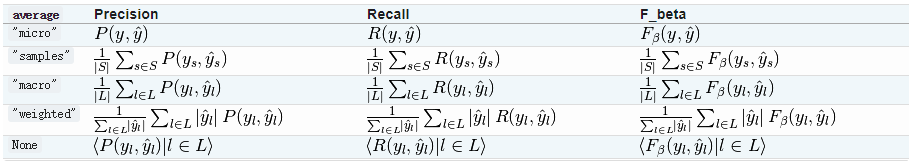from sklearn import metrics
y_true = [0, 1, 2, 0, 1, 2]
y_pred = [0, 2, 1, 0, 0, 1]
print metrics.precision_score(y_true, y_pred, average='macro')  # 输出：0.22
print metrics.recall_score(y_true, y_pred, average='micro')  # 输出：0.33
print metrics.f1_score(y_true, y_pred, average='weighted')  # 输出：0.26
print metrics.fbeta_score(y_true, y_pred, average='macro', beta=0.5)  # 输出：0.23
print metrics.precision_recall_fscore_support(y_true, y_pred, beta=0.5, average=None)  #输出：数组如下
# (array([ 0.66...,  0.        ,  0.        ]), array([ 1.,  0.,  0.]), array([ 0.71...,  0.        ,  0.        ]), array([2, 2, 2]...))

print metrics.recall_score(y_true, y_pred, labels=[1, 2], average='micro')  # 输出：0.0
print metrics.precision_score(y_true, y_pred, labels=[0, 1, 2, 3], average='macro')  # 输出：0.166..

Hinge损失

hinge_loss()函数使用hinge损失计算模型和数据之间的平均距离，这是仅考虑预测误差的单侧度量。 （Hinge损失用于最大化边缘分类器，如SVM支持向量机）如果标签用+1和-1编码，则y是真实值，w是由decision_function()输出的预测值，则hinge损失定义为：
LHingeyw=max{1wy0}=|1wy|+$L_ \text {Hinge}（y，w）= \max \left \{1 - wy，0 \right \} = \left | 1 - wy \right | _+$

LHingeywyt=max{1+ytyw0}$L_ \text {Hinge}（y_w，y_t）= \max \left \{1 + y_t - y_w，0 \right \}$

from sklearn import svm
from sklearn.metrics import hinge_loss
X = [, ]
y = [-1, 1]
est = svm.LinearSVC(random_state=0)
est.fit(X, y)  # 训练好线性分类器
pred_decision = est.decision_function([[-2], , [0.5]])
print pred_decision  # 输出预测值：array([-2.18...,  2.36...,  0.09...])
print hinge_loss([-1, 1, 1], pred_decision)  # 输出：0.3...

X = np.array([, , , ])
Y = np.array([0, 1, 2, 3])
labels = np.array([0, 1, 2, 3])
est = svm.LinearSVC()
est.fit(X, Y)
pred_decision = est.decision_function([[-1], , ])
y_true = [0, 2, 3]
hinge_loss(y_true, pred_decision, labels)   # 输出：0.56...

Log损失

log损失也称logistic回归损失或交叉熵损失，是一个建立在概率上的定义。它通常用于（多项式）逻辑回归和神经网络，以及期望最大化的一些变体中。
log损失是一个负值：
Llog(yp)=logPr(y|p)=(ylog(p)+(1y)log(1p))$L _ {\log}(y，p)= - \log\operatorname {Pr}(y | p) = - (y \log(p)+(1 - y)\log(1 - p))$

Llog(Y,P)=logPr(Y|P)=1NN1i=0K1k=0yi,klogpi,k$L_{\log}(Y, P) = \log\operatorname{Pr}(Y|P) = - \frac{1}{N} \sum_{i=0}^{N-1} \sum_{k=0}^{K-1} y_{i,k} \log p_{i,k}$

from sklearn.metrics import log_loss
y_true = [0, 0, 1, 1]
y_pred = [[.9, .1], [.8, .2], [.3, .7], [.01, .99]]
log_loss(y_true, y_pred)  # 输出：0.1738...

Matthews 相关系数

matthews_corrcoef函数计算二进制类的Matthews相关系数（MCC）。引用维基百科：

“The Matthews correlation coefficient is used in machine learning as a measure of the quality of binary (two-class) classifications. It takes into account true and false positives and negatives and is generally regarded as a balanced measure which can be used even if the classes are of very different sizes. The MCC is in essence a correlation coefficient value between -1 and +1. A coefficient of +1 represents a perfect prediction, 0 an average random prediction and -1 an inverse prediction. The statistic is also known as the phi coefficient.”

Matthews相关系数（MCC）用于机器学习，作为二分类质量的量度。它考虑到正例反例、判断对判断错，通常被认为是可以使用的平衡措施，即使类别大小极其不同。 MCC本质上是-1和+1之间的相关系数值。系数+1表示完美预测，0表示平均随机预测，-1表示反向预测。统计学也称为phi系数。

MCC=tp×tnfp×fn(tp+fp)(tp+fn)(tn+fp)(tn+fn)$MCC = \frac{tp \times tn - fp \times fn}{\sqrt{(tp + fp)(tp + fn)(tn + fp)(tn + fn)}}$.

• tk=KiCik$t_k=\sum_{i}^{K} C_{ik}$真正发生类k的次数，
• pk=KiCki$p_k=\sum_{i}^{K} C_{ki}$k类被预测的次数，
• c=KkCkk$c=\sum_{k}^{K} C_{kk}$正确预测的样本总数，
• s=KiKjCij$s=\sum_{i}^{K} \sum_{j}^{K} C_{ij}$样本总数。

MCC=c×sKkpk×tk(s2Kkp2k)×(s2Kkt2k)$MCC = \frac{ c \times s - \sum_{k}^{K} p_k \times t_k }{\sqrt{ (s^2 - \sum_{k}^{K} p_k^2) \times (s^2 - \sum_{k}^{K} t_k^2) }}$

from sklearn.metrics import matthews_corrcoef
y_true = [+1, +1, +1, -1]
y_pred = [+1, -1, +1, +1]
matthews_corrcoef(y_true, y_pred)  # 输出：-0.33

ROC

函数roc_curve()计算 receiver operating characteristic curve或者说是ROC曲线。 引用维基百科：

“A receiver operating characteristic (ROC), or simply ROC curve, is a graphical plot which illustrates the performance of a binary classifier system as its discrimination threshold is varied. It is created by plotting the fraction of true positives out of the positives (TPR = true positive rate) vs. the fraction of false positives out of the negatives (FPR = false positive rate), at various threshold settings. TPR is also known as sensitivity, and FPR is one minus the specificity or true negative rate.”

ROC或者是ROC曲线，是一个图形图，说明了二分类系统在鉴别阈值变化的情形下的性能。它通过在不同阈值设置下，TPR（正例中判对的概率）与FPR（被错判为正例的概率）。TPR又称为敏感度，FPR是1减去反例判对的概率。

import numpy as np
from sklearn.metrics import roc_curve
y = np.array([1, 1, 2, 2])
scores = np.array([0.1, 0.4, 0.35, 0.8])
fpr, tpr, thresholds = roc_curve(y, scores, pos_label=2)
print fpr  # 输出：[ 0.   0.5  0.5  1. ]
print tpr  # 输出：[ 0.5  0.5  1.   1. ]
print thresholds  # 输出：[ 0.8   0.4   0.35  0.1 ]

thresholds阈值的意义：所有的样本都要和这个阈值比较，如果大于这个阈值就是正例。所以，这个阈值越大，反例被错判为正例的可能性越小，当然，很多比较小的正例也会被忽略从而导致TPR也较小。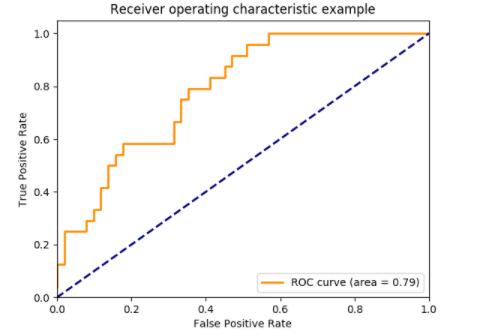roc_auc_score()函数计算ROC曲线下面积，也由AUC或AUROC表示。 通过计算roc曲线下的面积，曲线信息总结为一个数字。

import numpy as np
from sklearn.metrics import roc_auc_score
y_true = np.array([0, 0, 1, 1])
y_scores = np.array([0.1, 0.4, 0.35, 0.8])
roc_auc_score(y_true, y_scores)  # 输出：0.75

0-1损失

zero_one_loss()函数通过nsamples$n _ {\text {samples}}$计算0-1分类损失(L01)$(L_{0-1})$的和或平均值。 默认情况下，函数对样本进行标准化。 要获得L01$L_ {0-1}$的总和，将其normalize参数置为False。
在多标签分类中，如果所有预测标签与真实标签严格匹配，则zero_one_loss()为1，如果存在任何错误，则为0。 默认情况下，函数返回对子集不完全预测的百分比， 如果想让函数返回个数，设置normalize参数为False如果y^i$\hat {y} _i$是第i个样本的预测值，yi$y_i$是相应的真实值，则0-1损失L01$L_ {0-1}$定义为：
L01(yi,y^i)=1(y^iyi)$L_{0-1}(y_i, \hat{y}_i) = 1(\hat{y}_i \not= y_i)$

from sklearn.metrics import zero_one_loss
y_pred = [1, 2, 3, 4]
y_true = [2, 2, 3, 4]
print zero_one_loss(y_true, y_pred)  # 输出：0.25   表示预测错误的百分比是0.25
print zero_one_loss(y_true, y_pred, normalize=False)  # 输出：1  表示有1个预测错误
print zero_one_loss(np.array([[0, 1], [1, 1]]), np.ones((2, 2)))  # 输出：0.5
print zero_one_loss(np.array([[0, 1], [1, 1]]), np.ones((2, 2)),  normalize=False)  # 输出1

Brier score损失

brier_score_loss()函数计算二分类的Brier分数。引用维基百科：

The Brier score is a proper score function that measures the accuracy of probabilistic predictions. It is applicable to tasks in which predictions must assign probabilities to a set of mutually exclusive discrete outcomes.

Brier分数在用于衡量预测的准确性方面，是一个合适的评分函数。它适用于预测必须将概率分配给一组相互排斥的离散结果的任务。

该函数返回实际结果与可能结果的预测概率之间的均方差的得分。实际结果必须为1或0（真或假），而实际结果的预测概率可以是0到1之间的值。Brier损失也在0到1之间，分数越低（均方差越小），预测越准确。它可以被认为是对一组概率预测的“校准”的度量。
BS=1NNt=1(ftot)2$BS = \frac{1}{N} \sum_{t=1}^{N}(f_t - o_t)^2$

from sklearn.metrics import brier_score_loss
y_true = np.array([0, 1, 1, 0])
y_true_categorical = np.array(["spam", "ham", "ham", "spam"])
y_prob = np.array([0.1, 0.9, 0.8, 0.4])
y_pred = np.array([0, 1, 1, 0])
print brier_score_loss(y_true, y_prob)  # 输出；0.055
print brier_score_loss(y_true, 1-y_prob, pos_label=0)  # 输出；0.055
print brier_score_loss(y_true_categorical, y_prob, pos_label="ham")  # 输出；0.055
print brier_score_loss(y_true, y_prob > 0.5)  # 输出；0

多标签分级度量

在多标签学习中，每个样本可以具有与之相关的任何数量的真实标签，最后靠近真实标签的获得更高的分值和等级。

Coverage误差

coverage_error()函数计算包含在最终预测中的标签的平均数，以便预测所有真正的标签。如果您想知道有多少顶级评分标签，您必须平均预测，而不会丢失任何真正的标签，这很有用。因此，此指标的最好的情况（也就是最小值）是正确标签的平均数量。

给定一个二进制的真实标签矩阵，y {0,1}nsamples×nlabels$\in \left\{0, 1\right\}^{n_\text{samples} \times n_\text{labels}}$ ，和相应的分值f^Rnsamples×nlabels$\hat{f} \in \mathbb{R}^{n_\text{samples} \times n_\text{labels}}$，coverage被定义为：
coverage(y,f^)=1nsamplesnsamples1i=0maxj:yij=1rankij$coverage(y, \hat{f}) = \frac{1}{n_{\text{samples}}} \sum_{i=0}^{n_{\text{samples}} - 1} \max_{j:y_{ij} = 1} \text{rank}_{ij}$

import numpy as np
from sklearn.metrics import coverage_error
y_true = np.array([[1, 0, 0], [0, 0, 1]])
y_score = np.array([[0.75, 0.5, 1], [1, 0.2, 0.1]])
coverage_error(y_true, y_score)  # 输出：2.5

分级标签平均准确度

label_ranking_average_precision_score()函数实现标签rank的平均精度（LRAP）。该度量值与average_precision_score()函数相关，但是基于标签rank的信息，而不是准确率和召回率。
标签rank的平均精度（LRAP）是分配给每个样本的，rank一般较高真实标签对rank一般较低的总标签的比率的平均值。如果能够为每个样本相关标签提供更好的rank，这个指标就会产生更好的分数。获得的分数总是严格大于0，最佳值为1。如果每个样本只有一个相关标签，则标签排名平均精度等于mean reciprocal rank
给定一个二进制标签矩阵，即yRnsamples×nlabels$y \in \mathcal{R}^{n_\text{samples} \times n_\text{labels}}$，以及它的对应的预测rankf^Rnsamples×nlabels$\hat{f} \in \mathcal{R}^{n_\text{samples} \times n_\text{labels}}$，则平均精度定义为
LRAP(y,f^)=1nsamplesnsamples1i=01|yi|j:yij=1|Lij|rankij$LRAP(y, \hat{f}) = \frac{1}{n_{\text{samples}}}\sum_{i=0}^{n_{\text{samples}} - 1} \frac{1}{|y_i|}\sum_{j:y_{ij} = 1} \frac{|\mathcal{L}_{ij}|}{\text{rank}_{ij}}$

import numpy as np
from sklearn.metrics import label_ranking_average_precision_score
y_true = np.array([[1, 0, 0], [0, 0, 1]])
y_score = np.array([[0.75, 0.5, 1], [1, 0.2, 0.1]])
label_ranking_average_precision_score(y_true, y_score)  # 输出：0.416...

分级损失

label_ranking_loss()函数计算在样本上的排序错误（即正例的rank低于反例）的标签的rank损失的平均值（由正例和反例的倒数加权），最小值为0。
给定一个二进制标签矩阵y{0,1}nsamples×nlabels$y \in \left\{0, 1\right\}^{n_\text{samples} \times n_\text{labels}}$，相应的预测rank为f^Rnsamples×nlabels$\hat{f} \in \mathbb{R}^{n_\text{samples} \times n_\text{labels}}$，排名损失定义为：
ranking\_loss(y,f^)=1nsamplesnsamples1i=01|yi|(nlabels|yi|){(k,l):f^ik<f^il,yik=1,yil=0}$\text{ranking\_loss}(y, \hat{f}) = \frac{1}{n_{\text{samples}}}\sum_{i=0}^{n_{\text{samples}} - 1} \frac{1}{|y_i|(n_\text{labels} - |y_i|)}\left|\left\{(k, l): \hat{f}_{ik} < \hat{f}_{il}, y_{ik} = 1, y_{il} = 0 \right\}\right|$

import numpy as np
from sklearn.metrics import label_ranking_loss
y_true = np.array([[1, 0, 0], [0, 0, 1]])
y_score = np.array([[0.75, 0.5, 1], [1, 0.2, 0.1]])
label_ranking_loss(y_true, y_score)  # 输出：0.75
y_score = np.array([[1.0, 0.1, 0.2], [0.1, 0.2, 0.9]])  # 这样就能输出最小loss
label_ranking_loss(y_true, y_score)  # 输出：0.0

回归度量

sklearn.metrics模块实现了几个损失，评分和工具函数来衡量回归表现。其中一些已被增强以处理多输出情形：mean_squared_error()mean_absolute_error()explain_variance_score()r2_score()
这些函数具有一个multioutput关键字参数，用于指定平均每个目标的分数或损失的方式。默认值为'uniform_average'，它指定输出均匀加权均值。如果传递形如(n_outputs,)的数组，则将其解释为权重，并返回相应的加权平均值。如果指定了多重输出为'raw_values'，则所有未更改的单个分数或损失将以形状数组(n_outputs,)返回。
r2_score()interpret_variance_score()multioutput参数接受一个附加值'variance_weighted'。该选项通过相应目标变量的方差导出每个单独得分的加权。此设置量化了全局捕获的未缩放的方差。如果目标变量的scale范围不同，则该分数更好地解释较高的方差变量。对于向后兼容性，multioutput ='variance_weighted'r2_score()的默认值。将来会更改为uniform_average

Explained variance score

explain_variance_score()计算explained variance regression score.。

explained_variance(y,y^)=1Var{yy^}Var{y}$\texttt{explained_variance}(y, \hat{y}) = 1 - \frac{Var\{ y - \hat{y}\}}{Var\{y\}}$

from sklearn.metrics import explained_variance_score
y_true = [3, -0.5, 2, 7]
y_pred = [2.5, 0.0, 2, 8]
explained_variance_score(y_true, y_pred)  # 输出：0.957.。。
y_true = [[0.5, 1], [-1, 1], [7, -6]]
y_pred = [[0, 2], [-1, 2], [8, -5]]
print explained_variance_score(y_true, y_pred, multioutput='raw_values')  # 输出：[ 0.967...,  1.        ]
print explained_variance_score(y_true, y_pred, multioutput=[0.3, 0.7])  # 输出：0.990...

均值绝对误差

mean_absolute_error()函数计算平均绝对误差，对应于绝对误差损失或l1范数损失的预期值的风险度量。如果y^i$\hat {y} _i$是第i个样本的预测值，yi$y_i$是相应的真实值，则在nsamples$n _ {\text {samples}}$上估计的平均绝对误差（MAE）被定义为
MAE(y,y^)=1nsamplesnsamples1i=0|yiy^i|.$\text{MAE}(y, \hat{y}) = \frac{1}{n_{\text{samples}}} \sum_{i=0}^{n_{\text{samples}}-1} \left| y_i - \hat{y}_i \right|.$

from sklearn.metrics import mean_absolute_error
y_true = [3, -0.5, 2, 7]
y_pred = [2.5, 0.0, 2, 8]
mean_absolute_error(y_true, y_pred)  # 输出：0.5
y_true = [[0.5, 1], [-1, 1], [7, -6]]
y_pred = [[0, 2], [-1, 2], [8, -5]]
mean_absolute_error(y_true, y_pred)  # 输出：0.75
print mean_absolute_error(y_true, y_pred, multioutput='raw_values')  # 输出：【0.5， 1】
print mean_absolute_error(y_true, y_pred, multioutput=[0.3, 0.7])  # 输出：0.849...

均值平方误差

mean_squared_error()函数计算均方误差，与平方（二次）误差或损失的预期值对应的风险度量。如果y^i$\hat {y} _i$是第i个样本的预测值，yi$y_i$是相应的真实值，则在nsamples$n _ {\text {samples}}$上估计的均方误差（MSE）被定义为
MSE(y,y^)=1nsamplesnsamples1i=0(yiy^i)2.$\text{MSE}(y, \hat{y}) = \frac{1}{n_\text{samples}} \sum_{i=0}^{n_\text{samples} - 1} (y_i - \hat{y}_i)^2.$

from sklearn.metrics import mean_squared_error
y_true = [3, -0.5, 2, 7]
y_pred = [2.5, 0.0, 2, 8]
print mean_squared_error(y_true, y_pred)  # 输出：0.375
y_true = [[0.5, 1], [-1, 1], [7, -6]]
y_pred = [[0, 2], [-1, 2], [8, -5]]
print mean_squared_error(y_true, y_pred)  # 输出：0.7083

均值平方对数误差

mean_squared_log_error()函数计算对应于平方对数（二次）误差或损失的预期值的风险度量。如果y^i$\hat {y} _i$是第i个样本的预测值，yi$y_i$是相应的真实值，则在nsamples$n _ {\text {samples}}$上估计的均方对数误差（MSLE）被定义为
MSLE(y,y^)=1nsamplesnsamples1i=0(loge(1+yi)loge(1+y^i))2.$\text{MSLE}(y, \hat{y}) = \frac{1}{n_\text{samples}} \sum_{i=0}^{n_\text{samples} - 1} (\log_e (1 + y_i) - \log_e (1 + \hat{y}_i) )^2.$

from sklearn.metrics import mean_squared_log_error
y_true = [3, 5, 2.5, 7]
y_pred = [2.5, 5, 4, 8]
print mean_squared_log_error(y_true, y_pred)  # 输出：0.039.。

y_true = [[0.5, 1], [1, 2], [7, 6]]
y_pred = [[0.5, 2], [1, 2.5], [8, 8]]
print mean_squared_log_error(y_true, y_pred)  # 输出：0.044

中位数绝对误差

median_absolute_error()是非常有趣的，因为它可以减弱异常值的影响。 通过取目标和预测之间的所有绝对差值的中值来计算损失。如果y^i$\hat {y} _i$是第i个样本的预测值，yi$y_i$是相应的真实值，则在nsamples$n _ {\text {samples}}$上估计的中值绝对误差（MedAE）被定义为
MedAE(y,y^)=median(y1y^1,,yny^n)$\text{MedAE}(y, \hat{y}) = \text{median}(\mid y_1 - \hat{y}_1 \mid, \ldots, \mid y_n - \hat{y}_n \mid)$

median_absolute_error()不支持multioutput。

from sklearn.metrics import median_absolute_error
y_true = [3, -0.5, 2, 7]
y_pred = [2.5, 0.0, 2, 8]
median_absolute_error(y_true, y_pred)  # 输出：0.5

R2$R^{2}$ score，定义相关性

r2_score()函数计算R^2，即确定系数，可以表示特征模型对特征样本预测的好坏。 最佳分数为1.0，可以为负数（因为模型可能会更糟）。对于一个总是在预测y的期望值时不关注输入特征的连续模型，它的R^2分值是0.0。如果y^i$\hat {y} _i$是第i个样本的预测值，yi$y_i$是相应的真实值，则在nsamples$n _ {\text {samples}}$上估计的分数R^2被定义为
R2(y,y^)=1nsamples1i=0(yiy^i)2nsamples1i=0(yiy¯)2$R^2(y, \hat{y}) = 1 - \frac{\sum_{i=0}^{n_{\text{samples}} - 1} (y_i - \hat{y}_i)^2}{\sum_{i=0}^{n_\text{samples} - 1} (y_i - \bar{y})^2}$

from sklearn.metrics import r2_score
y_true = [3, -0.5, 2, 7]
y_pred = [2.5, 0.0, 2, 8]
print r2_score(y_true, y_pred)  # 0.948...

y_true = [[0.5, 1], [-1, 1], [7, -6]]
y_pred = [[0, 2], [-1, 2], [8, -5]]
print r2_score(y_true, y_pred, multioutput='variance_weighted')  # 0.938...

y_true = [[0.5, 1], [-1, 1], [7, -6]]
y_pred = [[0, 2], [-1, 2], [8, -5]]
print r2_score(y_true, y_pred, multioutput='uniform_average')  # 0.936...

print r2_score(y_true, y_pred, multioutput='raw_values')  # [ 0.965...,  0.908...]
print r2_score(y_true, y_pred, multioutput=[0.3, 0.7])  # 0.925...

聚类度量

sklearn.metrics模块实现了多种损失函数、评分函数和工具函数。 有关更多信息，请参阅集聚类性能评估部分

简陋评分器

在进行监督学习的过程中，简单清晰的检查包括将一个估计模型与简单的经验法则进行比较。 DummyClassifier实现了几种简单的分类策略：

• stratified根据训练数据类的分布产生随机数据。
• most_frequent总是将结果预测为训练集中最常用的标签。
• prior总是预测为先前最大的那个类。
• uniform产生均匀随机预测。
• constant总是预测为用户提供的常量标签。这种方法的主要靠的是F1分值，此时的正例数量较小。

from sklearn.datasets import load_iris
from sklearn.model_selection import train_test_split
X, y = iris.data, iris.target
y[y != 1] = -1
X_train, X_test, y_train, y_test = train_test_split(X, y, random_state=0)

from sklearn.dummy import DummyClassifier
from sklearn.svm import SVC
clf = SVC(kernel='linear', C=1).fit(X_train, y_train)
print clf.score(X_test, y_test)  # 0.63...

clf = DummyClassifier(strategy='most_frequent',random_state=0)
clf.fit(X_train, y_train)
print clf.score(X_test, y_test)  # 0.57...

clf = SVC(kernel='rbf', C=1).fit(X_train, y_train)
clf.score(X_test, y_test)  # 0.97...

我们看到准确率提高到近100％。建议使用交叉验证策略，以便更好地估计精度。更一般地说，当分类器的准确度太接近随机时，这可能意味着出现了一些问题：特征没有帮助，超参数没有正确调整，数据分布不平衡等
DummyRegressor还实现了四个简单的经验法则：

• mean：预测训练目标的平均值。
• median：预测训练目标的中位数。
• quantile：预测用户提供的分数量的训练目标。
• constant：预测用户提供的常数值。